Tutorials
data manipulation
+1

# Handling Categorical Data in Python

Learn the common tricks to handle categorical data and preprocess it to build machine learning models!If you are familiar with machine learning, you will probably have encountered categorical features in many datasets. These generally include different categories or levels associated with the observation, which are non-numerical and thus need to be converted so the computer can process them.

In this tutorial, you’ll learn the common tricks to handle this type of data and preprocess it to build machine learning models with them. More specifically, you will learn:

This tutorial covers the operations you have perform on categorical data before it can be used in an ML algorithm. But there is more to it. You will also have to clean your data. If you would like to know more about this process, be sure to take a look at DataCamp's Cleaning Data in Python course.

## Identifying Categorical Data: Nominal, Ordinal and Continuous

Categorical features can only take on a limited, and usually fixed, number of possible values. For example, if a dataset is about information related to users, then you will typically find features like country, gender, age group, etc. Alternatively, if the data you're working with is related to products, you will find features like product type, manufacturer, seller and so on.

These are all categorical features in your dataset. These features are typically stored as text values which represent various traits of the observations. For example, gender is described as Male (M) or Female (F), product type could be described as electronics, apparels, food etc.

Note that these type of features where the categories are only labeled without any order of precedence are called nominal features.

Features which have some order associated with them are called ordinal features. For example, a feature like economic status, with three categories: low, medium and high, which have an order associated with them.

There are also continuous features. These are numeric variables that have an infinite number of values between any two values. A continuous variable can be numeric or a date/time.

Regardless of what the value is used for, the challenge is determining how to use this data in the analysis because of the following constraints:

• Categorical features may have a very large number of levels, known as high cardinality, (for example, cities or URLs), where most of the levels appear in a relatively small number of instances.
• Many machine learning models, such as regression or SVM, are algebraic. This means that their input must be numerical. To use these models, categories must be transformed into numbers first, before you can apply the learning algorithm on them.
• While some ML packages or libraries might transform categorical data to numeric automatically based on some default embedding method, many other ML packages don’t support such inputs.
• For the machine, categorical data doesn’t contain the same context or information that humans can easily associate and understand. For example, when looking at a feature called City with three cities New York, New Jersey and New Delhi, humans can infer that New York is closely related to New Jersey as they are from same country, while New York and New Delhi are much different. But for the model, New York, New Jersey and New Delhi, are just three different levels (possible values) of the same feature City. If you don’t specify the additional contextual information, it will be impossible for the model to differentiate between highly different levels.

You therefore are faced with the challenge of figuring out how to turn these text values into numerical values for further processing and unmask lots of interesting information which these features might hide. Typically, any standard work-flow in feature engineering involves some form of transformation of these categorical values into numeric labels and then applying some encoding scheme on these values.

## General Exploration steps for Categorical Data

In this section, you'll focus on dealing with categorical features in the pnwflights14 dataset, but you can apply the same procedure to all kinds of datasets. pnwflights14 is a modified version of Hadley Wickham's nycflights13 dataset and contains information about all flights that departed from the two major airports of the Pacific Northwest (PNW), SEA in Seattle and PDX in Portland, in 2014: 162,049 flights in total.

To help understand what causes delays, it also includes a number of other useful datasets:

• weather: the hourly meterological data for each airport
• planes: constructor information about each plane
• airports: airport names and locations
• airlines: translation between two letter carrier codes and names

The datasets can be found here.

Since it's always a good idea to understand before starting working on it, you'll briefly explore the data! To do this, you will first import the basic libraries that you will be using throughout the tutorial, namely pandas, numpy and copy.

Also make sure that you set Matplotlib to plot inline, which means that the outputted plot will appear immediately under each code cell.

import pandas as pd
import numpy as np
import copy
%matplotlib inline


Next you will read the flights dataset in a pandas DataFrame with read_csv() and check the contents with the .head() method.

df_flights = pd.read_csv('https://raw.githubusercontent.com/ismayc/pnwflights14/master/data/flights.csv')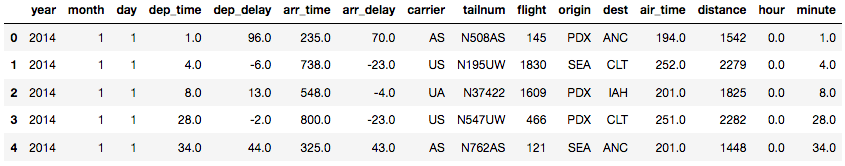As you will probably notice, the DataFrame above contains all kinds of information about flights like year, departure delay, arrival time, carrier, destination, etc.

Note if you are reading the RDS file formats you can do so by installing rpy2 library. Checkout this link to install the library on your system. The simplest way to install the library is using pip install rpy2 command on command line terminal.

Running the following code would read the flights.RDS file and load it in a pandas DataFrame. Remember that you already imported pandas earlier.

import rpy2.robjects as robjects
from rpy2.robjects import pandas2ri
pandas2ri.activate()
df_rds = pandas2ri.ri2py(df_rds)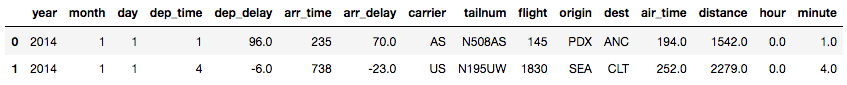The same rpy2 library can also be used to read rda file formats. The code below reads and loads flights.rda into a pandas DataFrame:

from rpy2.robjects import r
import rpy2.robjects.pandas2ri as pandas2ri
df_rda=pandas2ri.ri2py_dataframe(r[rf])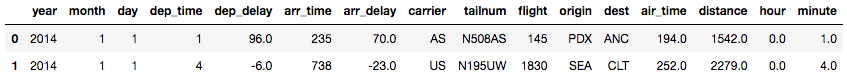The next step is to gather some information about different column in your DataFrame. You can do so by using .info(), which basically gives you information about the number of rows, columns, column data types, memory usage, etc.

print(df_flights.info())

<class 'pandas.core.frame.DataFrame'>
RangeIndex: 162049 entries, 0 to 162048
Data columns (total 16 columns):
year         162049 non-null int64
month        162049 non-null int64
day          162049 non-null int64
dep_time     161192 non-null float64
dep_delay    161192 non-null float64
arr_time     161061 non-null float64
arr_delay    160748 non-null float64
carrier      162049 non-null object
tailnum      161801 non-null object
flight       162049 non-null int64
origin       162049 non-null object
dest         162049 non-null object
air_time     160748 non-null float64
distance     162049 non-null int64
hour         161192 non-null float64
minute       161192 non-null float64
dtypes: float64(7), int64(5), object(4)
memory usage: 19.8+ MB
None


As you can see, columns like year, month and day are read as integers, and dep_time, dep_delay etc. are read as floats.

The columns with object dtype are the possible categorical features in your dataset.

The reason why you would say that these categorical features are 'possible' is because you shouldn't not completely rely on .info() to get the real data type of the values of a feature, as some missing values that are represented as strings in a continuous feature can coerce it to read them as object dtypes.

That's why it's always a good idea to investigate your raw dataset thoroughly and then think about cleaning it.

One of the most common ways to analyze the relationship between a categorical feature and a continuous feature is to plot a boxplot. The boxplot is a simple way of representing statistical data on a plot in which a rectangle is drawn to represent the second and third quartiles, usually with a vertical line inside to indicate the median value. The lower and upper quartiles are shown as horizontal lines at either side of the rectangle.

You can plot a boxplot by invoking .boxplot() on your DataFrame. Here, you will plot a boxplot of the dep_time column with respect to the two origin of the flights from PDX and SEA.

df_flights.boxplot('dep_time','origin',rot = 30,figsize=(5,6))

<matplotlib.axes._subplots.AxesSubplot at 0x7f32ee10f550>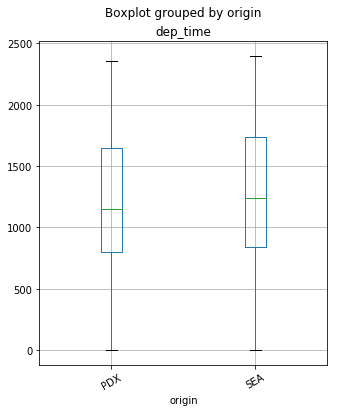As you will only be dealing with categorical features in this tutorial, it's better to filter them out. You can create a separate DataFrame consisting of only these features by running the following command. The method .copy() is used here so that any changes made in new DataFrame don't get reflected in the original one.

cat_df_flights = df_flights.select_dtypes(include=['object']).copy()


Again, use the .head() method to check if you have filtered the required columns.

cat_df_flights.head()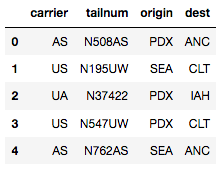One of the most common data pre-processing steps is to check for null values in the dataset. You can get the total number of missing values in the DataFrame by the following one liner code:

print(cat_df_flights.isnull().values.sum())

248


Let's also check the column-wise distribution of null values:

print(cat_df_flights.isnull().sum())

carrier      0
tailnum    248
origin       0
dest         0
dtype: int64


It seems that only the tailnum column has null values. You can do a mode imputation for those null values. The function fillna() is handy for such operations.

Note the chaining of method .value_counts() in the code below. This returns the frequency distribution of each category in the feature, and then selecting the top category, which is the mode, with the .index attribute.

cat_df_flights = cat_df_flights.fillna(cat_df_flights['tailnum'].value_counts().index)


Let's check the number of null values after imputation should result in a zero count.

print(cat_df_flights.isnull().values.sum())

0


Another Exploratory Data Analysis (EDA) step that you might want to do on categorical features is the frequency distribution of categories within the feature, which can be done with the .value_counts() method as described earlier.

print(cat_df_flights['carrier'].value_counts())

AS    62460
WN    23355
OO    18710
DL    16716
UA    16671
AA     7586
US     5946
B6     3540
VX     3272
F9     2698
HA     1095
Name: carrier, dtype: int64


To know the count of distinct categories within the feature you can chain the previous code with the .count() method:

print(cat_df_flights['carrier'].value_counts().count())

11


Visual exploration is the most effective way to extract information between variables.

Below is a basic template to plot a barplot of the frequency distribution of a categorical feature using the seaborn package, which shows the frequency distribution of the carrier column. You can play with different arguments to change the look of the plot. If you want to learn more about seaborn, you can take a look at this tutorial.

%matplotlib inline
import seaborn as sns
import matplotlib.pyplot as plt
carrier_count = cat_df_flights['carrier'].value_counts()
sns.set(style="darkgrid")
sns.barplot(carrier_count.index, carrier_count.values, alpha=0.9)
plt.title('Frequency Distribution of Carriers')
plt.ylabel('Number of Occurrences', fontsize=12)
plt.xlabel('Carrier', fontsize=12)
plt.show()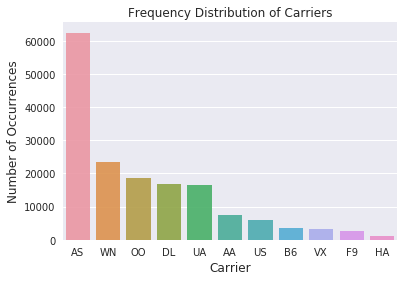Similarly, you could plot a pie chart with the matplotlib library to get the same information. The labels list below holds the category names from the carrier column:

labels = cat_df_flights['carrier'].astype('category').cat.categories.tolist()
counts = cat_df_flights['carrier'].value_counts()
sizes = [counts[var_cat] for var_cat in labels]
fig1, ax1 = plt.subplots()
ax1.pie(sizes, labels=labels, autopct='%1.1f%%', shadow=True) #autopct is show the % on plot
ax1.axis('equal')
plt.show()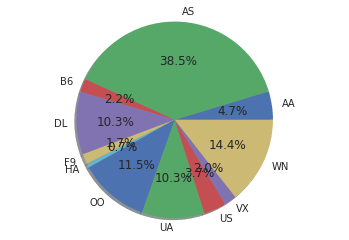## Encoding Categorical Data

You will now learn different techniques to encode the categorical features to numeric quantities. To keep it simple, you will apply these encoding methods only on the carrier column. However, the same approach can be extended to all columns.

The techniques that you'll cover are the following:

• Replacing values
• Encoding labels
• One-Hot encoding
• Binary encoding
• Backward difference encoding
• Miscellaneous features

### Replace Values

Let's start with the most basic method, which is just replacing the categories with the desired numbers. This can be achieved with the help of the replace() function in pandas. The idea is that you have the liberty to choose whatever numbers you want to assign to the categories according to the business use case.

You will now create a dictionary which contains mapping numbers for each category in the carrier column:

replace_map = {'carrier': {'AA': 1, 'AS': 2, 'B6': 3, 'DL': 4,
'F9': 5, 'HA': 6, 'OO': 7 , 'UA': 8 , 'US': 9,'VX': 10,'WN': 11}}


Note that defining a mapping via a hard coded dictionary is easy when the number of categories is low, like in this case which is 11. You can achieve the same mapping with the help of dictionary comprehensions as shown below. This will be useful when the categories count is high and you don't want to type out each mapping. You will store the category names in a list called labels and then zip it to a seqeunce of numbers and iterate over it.

labels = cat_df_flights['carrier'].astype('category').cat.categories.tolist()
replace_map_comp = {'carrier' : {k: v for k,v in zip(labels,list(range(1,len(labels)+1)))}}

print(replace_map_comp)

{'carrier': {'AA': 1, 'OO': 7, 'DL': 4, 'F9': 5, 'B6': 3, 'US': 9, 'AS': 2, 'WN': 11, 'VX': 10, 'HA': 6, 'UA': 8}}


Throughout this tutorial, you will be making a copy of the dataset via the .copy() method to practice each encoding technique to ensure that the original DataFrame stays intact and whatever changes you are doing happen only in the copied one.

cat_df_flights_replace = cat_df_flights.copy()


Use the replace() function on the DataFrame by passing the mapping dictionary as argument:

cat_df_flights_replace.replace(replace_map_comp, inplace=True)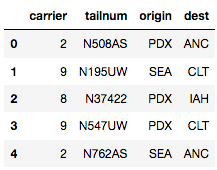As you can observe, you have encoded the categories with the mapped numbers in your DataFrame.

You can also check the dtype of the newly encoded column, which is now converted to integers.

print(cat_df_flights_replace['carrier'].dtypes)

int64


Tip: in Python, it's a good practice to typecast categorical features to a category dtype because they make the operations on such columns much faster than the object dtype. You can do the typecasting by using .astype() method on your columns like shown below:

cat_df_flights_lc = cat_df_flights.copy()
cat_df_flights_lc['carrier'] = cat_df_flights_lc['carrier'].astype('category')
cat_df_flights_lc['origin'] = cat_df_flights_lc['origin'].astype('category')

print(cat_df_flights_lc.dtypes)

carrier    category
tailnum      object
origin     category
dest         object
dtype: object


You can validate the faster operation of the category dtype by timing the execution time of the same operation done on a DataFrame with columns as category dtype and object dtype by using the time library.

Let's say you want to calculate the number of flights for each carrier from each origin places, you can use the .groupby() and .count() methods on your DataFrame to do so.

import time
%timeit cat_df_flights.groupby(['origin','carrier']).count() #DataFrame with object dtype columns

10 loops, best of 3: 28.6 ms per loop

%timeit cat_df_flights_lc.groupby(['origin','carrier']).count() #DataFrame with category dtype columns

10 loops, best of 3: 20.1 ms per loop


Note that the DataFrame with category dtype is much faster.

### Label Encoding

Another approach is to encode categorical values with a technique called "label encoding", which allows you to convert each value in a column to a number. Numerical labels are always between 0 and n_categories-1.

You can do label encoding via attributes .cat.codes on your DataFrame's column.

cat_df_flights_lc['carrier'] = cat_df_flights_lc['carrier'].cat.codes

cat_df_flights_lc.head() #alphabetically labeled from 0 to 10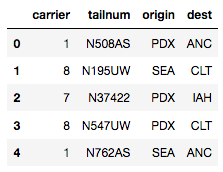Sometimes, you might just want to encode a bunch of categories within a feature to some numeric value and encode all the other categories to some other numeric value.

You could do this by using numpy's where() function like shown below. You will encode all the US carrier flights to value 1 and other carriers to value 0. This will create a new column in your DataFrame with the encodings. Later, if you want to drop the original column, you can do so by using the drop() function in pandas.

cat_df_flights_specific = cat_df_flights.copy()
cat_df_flights_specific['US_code'] = np.where(cat_df_flights_specific['carrier'].str.contains('US'), 1, 0)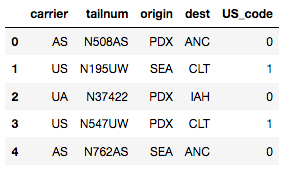You can achieve the same label encoding using scikit-learn's LabelEncoder:

cat_df_flights_sklearn = cat_df_flights.copy()

from sklearn.preprocessing import LabelEncoder

lb_make = LabelEncoder()
cat_df_flights_sklearn['carrier_code'] = lb_make.fit_transform(cat_df_flights['carrier'])

cat_df_flights_sklearn.head() #Results in appending a new column to df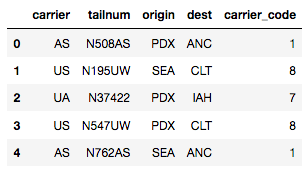Label encoding is pretty much intuitive and straight-forward and may give you a good performance from your learning algorithm, but it has as disadvantage that the numerical values can be misinterpreted by the algorithm. Should the carrier US (encoded to 8) be given 8x more weight than the carrier AS (encoded to 1) ?

To solve this issue there is another popular way to encode the categories via something called one-hot encoding.

### One-Hot encoding

The basic strategy is to convert each category value into a new column and assign a 1 or 0 (True/False) value to the column. This has the benefit of not weighting a value improperly.

There are many libraries out there that support one-hot encoding but the simplest one is using pandas' .get_dummies() method.

This function is named this way because it creates dummy/indicator variables (1 or 0). There are mainly three arguments important here, the first one is the DataFrame you want to encode on, second being the columns argument which lets you specify the columns you want to do encoding on, and third, the prefix argument which lets you specify the prefix for the new columns that will be created after encoding.

cat_df_flights_onehot = cat_df_flights.copy()
cat_df_flights_onehot = pd.get_dummies(cat_df_flights_onehot, columns=['carrier'], prefix = ['carrier'])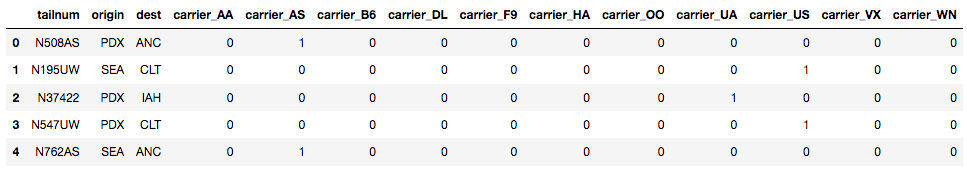As you can see, the column carrier_AS gets value 1 at the 0th and 4th observation points as those points had the AS category labeled in the original DataFrame. Likewise for other columns also.

scikit-learn also supports one hot encoding via LabelBinarizer and OneHotEncoder in its preprocessing module (check out the details here). Just for the sake of practicing you will do the same encoding via LabelBinarizer:

cat_df_flights_onehot_sklearn = cat_df_flights.copy()

from sklearn.preprocessing import LabelBinarizer

lb = LabelBinarizer()
lb_results = lb.fit_transform(cat_df_flights_onehot_sklearn['carrier'])
lb_results_df = pd.DataFrame(lb_results, columns=lb.classes_)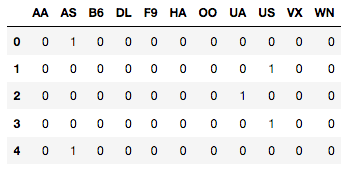Note that this lb_results_df resulted in a new DataFrame with only the one hot encodings for the feature carrier. This needs to be concatenated back with the original DataFrame, which can be done via pandas' .concat() method. The axis argument is set to 1 as you want to merge on columns.

result_df = pd.concat([cat_df_flights_onehot_sklearn, lb_results_df], axis=1)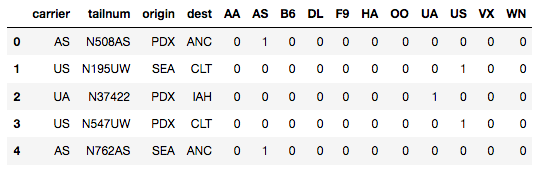While one-hot encoding solves the problem of unequal weights given to categories within a feature, it is not very useful when there are many categories, as that will result in formation of as many new columns, which can result in the curse of dimensionality. The concept of the “curse of dimensionality” discusses that in high-dimensional spaces some things just stop working properly.

### Binary Encoding

This technique is not as intuitive as the previous ones. In this technique, first the categories are encoded as ordinal, then those integers are converted into binary code, then the digits from that binary string are split into separate columns. This encodes the data in fewer dimensions than one-hot.

You can do binary encoding via a number of ways but the simplest one is using the category_encoders library. You can install category_encoders via pip install category_encoders on cmd or just download and extract the .tar.gz file from the site.

You have to first import the category_encoders library after installing it. Invoke the BinaryEncoder function by specifying the columns you want to encode and then call the .fit_transform() method on it with the DataFrame as the argument.

cat_df_flights_ce = cat_df_flights.copy()

import category_encoders as ce

encoder = ce.BinaryEncoder(cols=['carrier'])
df_binary = encoder.fit_transform(cat_df_flights_ce)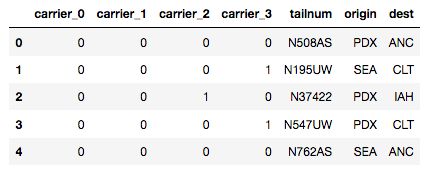Notice that four new columns are created in place of the carrier column with binary encoding for each category in the feature.

Note that category_encoders is a very useful library for encoding categorical columns. Not only does it support one-hot, binary and label encoding, but also other advanced encoding methods like Helmert contrast, polynomial contrast, backward difference, etc.

### 5. Backward Difference Encoding

This technique falls under the contrast coding system for categorical features. A feature of K categories, or levels, usually enters a regression as a sequence of K-1 dummy variables. In backward difference coding, the mean of the dependent variable for a level is compared with the mean of the dependent variable for the prior level. This type of coding may be useful for a nominal or an ordinal variable.

If you want to learn other contrast coding methods you can check out this resource.

The code structure is pretty much the same as any method in the category_encoders library, just this time you will call BackwardDifferenceEncoder from it:

encoder = ce.BackwardDifferenceEncoder(cols=['carrier'])
df_bd = encoder.fit_transform(cat_df_flights_ce)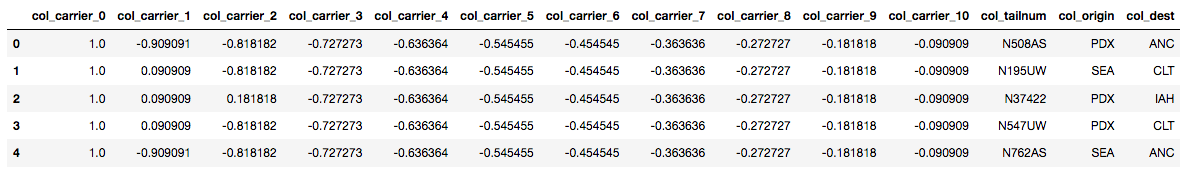The interesting thing here is that you can see that the results are not the standard 1’s and 0’s you saw in the dummy encoding examples but rather regressed continuous values.

### Miscellaneous Features

Sometimes you may encounter categorical feature columns which specify the ranges of values for observation points, for example, the age column might be described in the form of categories like 0-20, 20-40 and so on.

While there can be a lot of ways to deal with such features, the most common ones are either split these ranges into two separate columns or replace them with some measure like the mean of that range.

You will first create a dummy DataFrame which has just one feature age with ranges specified using the pandas DataFrame function. Then you will split the column on the delimeter - into two columns start and end using split() with a lambda() function. If you want to learn more about lambda functions, check out this tutorial.

dummy_df_age = pd.DataFrame({'age': ['0-20', '20-40', '40-60','60-80']})
dummy_df_age['start'], dummy_df_age['end'] = zip(*dummy_df_age['age'].map(lambda x: x.split('-')))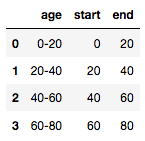To replace the range with its mean, you will write a split_mean() function which basically takes one range at a time, splits it, then calculates the mean and returns it. To apply a certain function to all the entities of a column you will use the .apply() method:

dummy_df_age = pd.DataFrame({'age': ['0-20', '20-40', '40-60','60-80']})

def split_mean(x):
split_list = x.split('-')
mean = (float(split_list)+float(split_list))/2
return mean

dummy_df_age['age_mean'] = dummy_df_age['age'].apply(lambda x: split_mean(x))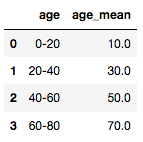## Dealing with Categorical Features in Big Data with Spark

Now you will learn how to read a dataset in Spark and encode categorical variables in Apache Spark's Python API, Pyspark. But before that it's good to brush up on some basic knowledge about Spark.

Spark is a platform for cluster computing. It lets you spread data and computations over clusters with multiple nodes. Splitting up your data makes it easier to work with very large datasets because each node only works with a small amount of data.

As each node works on its own subset of the total data, it also carries out a part of the total calculations required, so that both data processing and computations are performed in parallel over the nodes in the cluster.

Deciding whether or not Spark is the best solution for your problem takes some experience, but you can consider questions like:

• Is my data too big to work with on a single machine?
• Can my calculations be easily parallelized?

The first step in using Spark is connecting to a cluster. In practice, the cluster will be hosted on a remote machine that's connected to all other nodes. There will be one computer, called the master that manages splitting up the data and the computations. The master is connected to the rest of the computers in the cluster, which are called slaves. The master sends the slaves data and calculations to run, and they send their results back to the master.

When you're just getting started with Spark, it's simpler to just run a cluster locally. If you wish to run Spark on a cluster and use Jupyter Notebook, you can check out this blog.

If you wish to learn more about Spark, check out this great tutorial which covers almost everything about it, or DataCamp's Introduction to PySpark course.

The first step in Spark programming is to create a SparkContext. SparkContext is required when you want to execute operations in a cluster. SparkContext tells Spark how and where to access a cluster. You'll start by importing SparkContext.

from pyspark import SparkContext
sc = SparkContext()


Note that if you are working on Spark's interactive shell then you don't have to import SparkContext as it will already be in your environment as sc.

To start working with Spark DataFrames, you first have to create a SparkSession object from your SparkContext. You can think of the SparkContext as your connection to the cluster and the SparkSession as your interface with that connection.

Note that if you are working in Spark's interactive shell you'll have a SparkSession called spark available in your workspace!

from pyspark.sql import SparkSession as spark


Once you've created a SparkSession, you can start poking around to see what data is in your cluster.

Your SparkSession has an attribute called catalog which lists all the data inside the cluster. This attribute has a few methods for extracting different pieces of information.

One of the most useful is the .listTables() method, which returns the names of all the tables in your cluster as a list.

print(spark.catalog.listTables())

[]


You will now load the flights dataset in the Spark DataFrame.

To read a .csv file and create a Spark DataFrame you can use the .read attribute of your SparkSession object. Here, apart from reading the csv file, you have to additionally specify the headers option to be True, since you have column names in the dataset. Also, the inferSchema argument is set to True, which basically peeks at the first row of the data to determine the fields' names and types.

spark_flights = spark.read.format("csv").option('header',True).load('Downloads/datasets/nyc_flights/flights.csv',inferSchema=True)


To check the contents of your DataFrame you can run the .show() method on the DataFrame.

spark_flights.show(3)

+----+-----+---+--------+---------+--------+---------+-------+-------+------+------+----+--------+--------+----+------+
|year|month|day|dep_time|dep_delay|arr_time|arr_delay|carrier|tailnum|flight|origin|dest|air_time|distance|hour|minute|
+----+-----+---+--------+---------+--------+---------+-------+-------+------+------+----+--------+--------+----+------+
|2014|    1|  1|       1|       96|     235|       70|     AS| N508AS|   145|   PDX| ANC|     194|    1542|   0|     1|
|2014|    1|  1|       4|       -6|     738|      -23|     US| N195UW|  1830|   SEA| CLT|     252|    2279|   0|     4|
|2014|    1|  1|       8|       13|     548|       -4|     UA| N37422|  1609|   PDX| IAH|     201|    1825|   0|     8|
+----+-----+---+--------+---------+--------+---------+-------+-------+------+------+----+--------+--------+----+------+
only showing top 3 rows


If you wish to convert a pandas DataFrame to a Spark DataFrame, use the .createDataFrame() method on your SparkSession object with the DataFrame's name as argument.

To have a look at the schema of the DataFrame you can invoke .printSchema() as follows:

spark_flights.printSchema()

root
|-- year: integer (nullable = true)
|-- month: integer (nullable = true)
|-- day: integer (nullable = true)
|-- dep_time: string (nullable = true)
|-- dep_delay: string (nullable = true)
|-- arr_time: string (nullable = true)
|-- arr_delay: string (nullable = true)
|-- carrier: string (nullable = true)
|-- tailnum: string (nullable = true)
|-- flight: integer (nullable = true)
|-- origin: string (nullable = true)
|-- dest: string (nullable = true)
|-- air_time: string (nullable = true)
|-- distance: integer (nullable = true)
|-- hour: string (nullable = true)
|-- minute: string (nullable = true)


Note that Spark doesn't always guess the data type of the columns right and you can see that some of the columns (arr_delay, air_time, etc.) which seem to have numeric values are read as strings rather than integers or floats, due to the presence of missing values.

At this point, if you check the data in your cluster using the .catalog attribute and the .listTables() method like you did before, you will find it's still empty. This is because you DataFrame is currently stored locally, not in the SparkSession catalog.

To access the data in this way, you have to save it as a temporary table. You can do so by using the .createOrReplaceTempView() method. This method registers the DataFrame as a table in the catalog, but as this table is temporary, it can only be accessed from the specific SparkSession used to create the Spark DataFrame.

spark_flights.createOrReplaceTempView("flights_temp")


Print the tables in catalog again:

print(spark.catalog.listTables())

[Table(name=u'flights_temp', database=None, description=None, tableType=u'TEMPORARY', isTemporary=True)]


Now you have registered the flight_temp table as a temporary table in your catalog.

Now that you have gotten your hands dirty with a little bit of PySpark code, it's time to see how to encode categorical features. To keep things neat, you will create a new DataFrame which consists of only the carrier column by using the .select() method.

carrier_df = spark_flights.select("carrier")
carrier_df.show(5)

+-------+
|carrier|
+-------+
|     AS|
|     US|
|     UA|
|     US|
|     AS|
+-------+
only showing top 5 rows


The two most common ways to encode categorical features in Spark are using StringIndexer and OneHotEncoder.

• StringIndexer encodes a string column of labels to a column of label indices. The indices are in [0, numLabels] ordered by label frequencies, so the most frequent label gets index 0. This is similar to label encoding in pandas.

You will start by importing the StringIndexer class from the pyspark.ml.feature module. The main arguments inside StringIndexer are inputCol and outputCol, which are self-explanatory. After you create the StringIndex object you call the .fit() and .transform() methods with the DataFrame as the argument passed as shown:

from pyspark.ml.feature import StringIndexer
carr_indexer = StringIndexer(inputCol="carrier",outputCol="carrier_index")
carr_indexed = carr_indexer.fit(carrier_df).transform(carrier_df)

carr_indexed.show(7)

+-------+-------------+
|carrier|carrier_index|
+-------+-------------+
|     AS|          0.0|
|     US|          6.0|
|     UA|          4.0|
|     US|          6.0|
|     AS|          0.0|
|     DL|          3.0|
|     UA|          4.0|
+-------+-------------+
only showing top 7 rows


Since AS was the most frequent category in the carrier column, it got the index 0.0.

• OneHotEncoder: as you already read before, one-hot encoding maps a categorical feature, represented as a label index, to a binary vector with at most a single one-value indicating the presence of a specific feature value from among the set of all feature values.

For example, with 5 categories, an input value of 2.0 would map to an output vector of [0.0, 0.0, 1.0, 0.0]. The last category is not included by default (configurable via OneHotEncoder .dropLast because it makes the vector entries sum up to one, and hence linearly dependent. That means that an input value of 4.0 would map to [0.0, 0.0, 0.0, 0.0].

Note that this is different from scikit-learn's OneHotEncoder, which keeps all categories. The output vectors are sparse.

For a string type like in this case, it is common to encode features using StringIndexer first, here carrier_index. Then pass that column to the OneHotEncoder class.

The code is shown below.

carrier_df_onehot = spark_flights.select("carrier")

from pyspark.ml.feature import OneHotEncoder, StringIndexer

stringIndexer = StringIndexer(inputCol="carrier", outputCol="carrier_index")
model = stringIndexer.fit(carrier_df_onehot)
indexed = model.transform(carrier_df_onehot)
encoder = OneHotEncoder(dropLast=False, inputCol="carrier_index", outputCol="carrier_vec")
encoded = encoder.transform(indexed)

encoded.show(7)

+-------+-------------+--------------+
|carrier|carrier_index|   carrier_vec|
+-------+-------------+--------------+
|     AS|          0.0|(11,,[1.0])|
|     US|          6.0|(11,,[1.0])|
|     UA|          4.0|(11,,[1.0])|
|     US|          6.0|(11,,[1.0])|
|     AS|          0.0|(11,,[1.0])|
|     DL|          3.0|(11,,[1.0])|
|     UA|          4.0|(11,,[1.0])|
+-------+-------------+--------------+
only showing top 7 rows


Note that OneHotEncoder has created a vector for each category which can then be processed further by your machine learning pipeline.

There are some more methods available in Spark like VectorIndexer, but you have already mastered the most popular ones. If you wish to explore more, check out Spark's fantastic documentation.

## Conclusion

Hurray!! You have come a long way! You have explored most of the bits and pieces which are out there about dealing with categorical features in the machine learning realm. You started with basic EDA in pandas and then you practiced the different encoding methods available. You also learned a bit about Spark's architecture and moved to encoding categorical data in PySpark. That's a lot, but there is always so much more to learn. You will also have to make sure that you data is properly cleaned. To learn how to do so, take a look at our Cleaning Data in Python course. Happy exploring!!